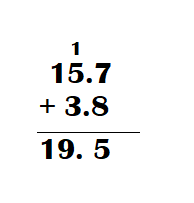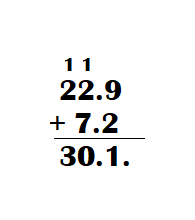Practice the problems of Math in Focus Grade 4 Workbook Answer Key Chapter 8 Practice 1 Adding Decimals to score better marks in the exam.

Fill in the blanks. Write each sum as a decimal.

Example
0.3 + 0.5 = 3 tenths + 5 tenths
= 8 tenths
= 0.8

Question 1.
0.8 + 0.2 = ___ tenths + ___ tenths
= ____ tenths
= ____
0.8 + 0.2 = 0.10.

Explanation:
0.8 + 0.2 = 8 tenths + 2 tenths
= 10 tenths
= 1.0.

Question 2.
0.7 + 0.7 = ___ tenths + ___ tenths
= ____ tenths
= ____
0.7 + 0.7 = 1.4.

Explanation:
0.7 + 0.7 = 7 tenths + 7 tenths
= 14 tenths
= 1.4.

Question 3.
0.9 + 0.8 = ___ tenths + ___ tenths
= ____ tenths
= ____
0.9 + 0.8 = 1.7.

Explanation:
0.9 + 0.8 = 9 tenths + 8 tenths
= 17 tenths
= 1.7.

Fill in the blanks.
Question 4.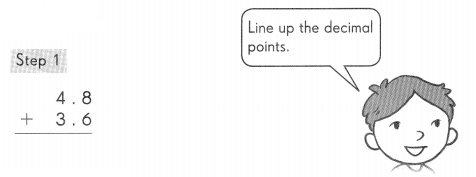8 tenths + 6 tenths = ___  tenths
Regroup the tenths.
___ tenths = __ one and __ tenths.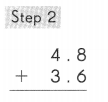4 ones + 3 ones + ____ one = ___ ones
So, 4.8 + 3.6 =

4.8 + 3.6 = 8.4.

Explanation:8 tenths + 6 tenths = 14 tenths
Regroup the tenths.
14 tenths = 1 one and 4 tenths.4 ones + 3 ones + 1 one = 8 ones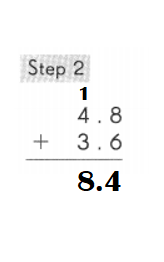Question 5.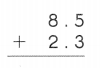8.5 + 2.3 = 10.8.

Explanation:
Step 1:5 tenths + 3 tenths = 8 tenths
Step 2:8 ones + 2 ones  = 10 ones.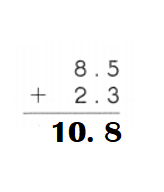Question 6.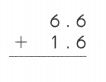6.6 + 1.6 = 8.2.

Explanation:6 tenths + 6 tenths = 12 tenths.
Regroup the tenths.
12 tenths = 1 one and 2 tenths.6 ones + 1 ones + 1 one = 8 ones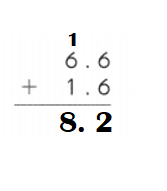Write in vertical form. Then add.
Question 7.
15.7 + 3.8 = ___